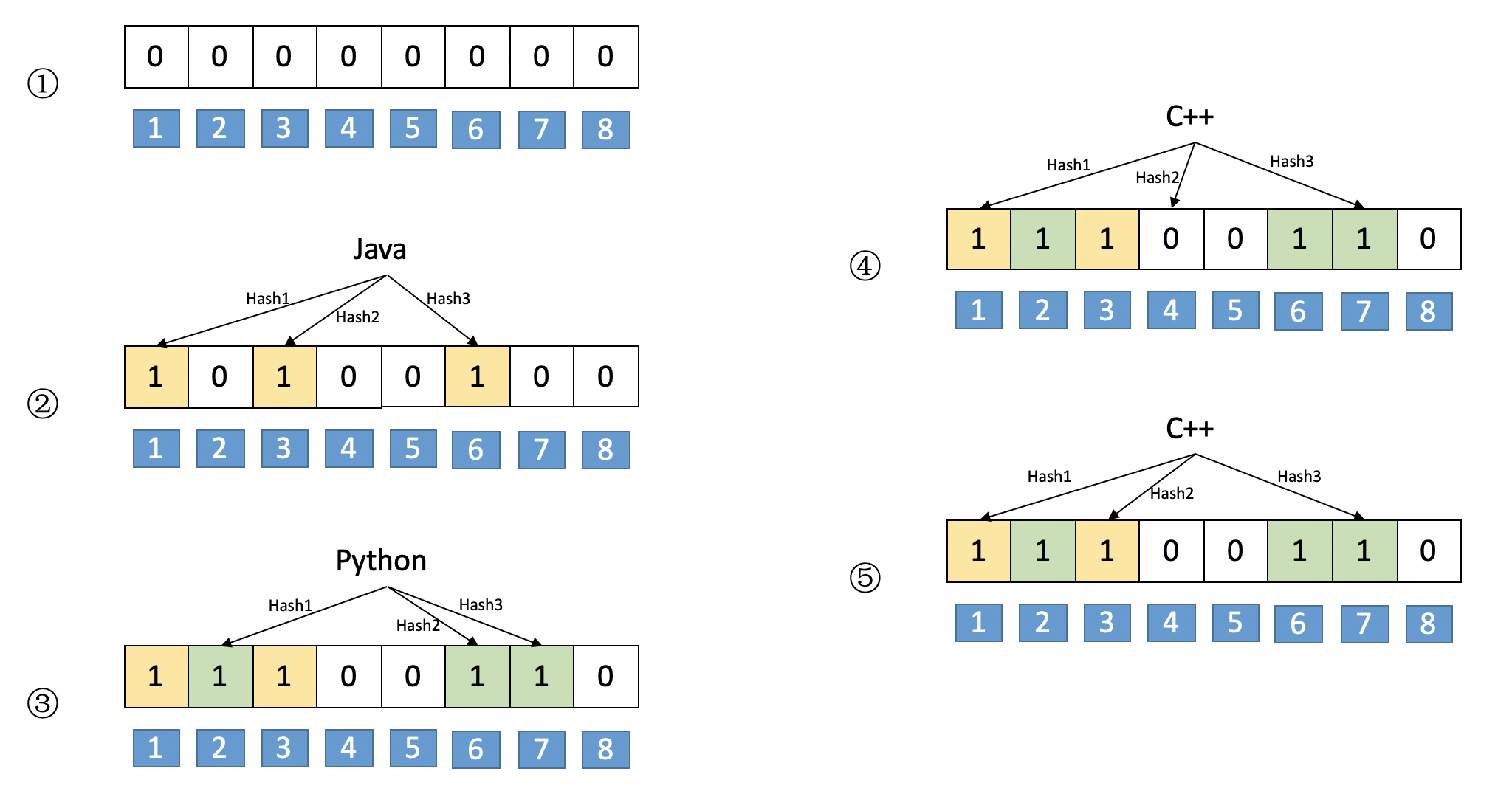# [布隆过滤器BloomFilter] 举例说明+证明推导

### 二、布隆过滤器功能

• 用于解决去重问题
• 起到去重的同时，空间上能节省90%以上
• 会有很小的误判率（False positive），即BloomFilter判断为不存在的一定不存在，但是其判定为存在也可能不存在（请参见原理部分，更容易理解）

### 三、Bloom Filter思想

#### 2、举例说明①如上图所示，第①步是布隆过滤器的初始结果，在这一步中，所以bit位都初始化为0
②现在如果有一个词“Java”，其hash值为1，3，6，则将其对应的bit位置1，如图②所示
③现在有另外一个词“Python”，其hash值为2，6，7，则将其对应的bit位置1，如果③所示，注意：6位置的1被覆盖
④如果现在需要查询“C++”是否存在，若“C++”对应的hash值是1，4，7，则bit为1和7对应元素是1，但是bit4位置元素是0，所以可以判断“C++”现在不存在。

⑤如果现在需要查询“C++”是否存在，若“C++”对应的hash值是1，6，7，则hash位置对应的元素都是1，所以可以判断“C++”可能存在。

#### 3、BloomFilter可以支持的操作

• 支持插入操作
从上述例子可以知道，如果想要插入一个元素到布隆过滤器，只需要将其对应hash对应元素置1
• 支持查询操作
上述例子中已经提到，可以支持查询操作，也就是判断其是否已经存在。当需要查询的词的所有bit位所有都为1时，说明待查询词可能已经存在。但是只要有一个为0，则可以说明，待查询词一定不存在。
• 支持删除操作么？
原则上BloomFilter不支持删除操作，因为其置1操作是覆盖式的，如果现在需要删除“Python”这个词，则需要将其对应的hash值位置恢复为0，即将2，6，7位置恢复为0，这种方法会使得“Java”中的6位置处的1失踪。
当然可以采用一种方法就是计数法，比如现在BloomFilter中6位置处已经为1，再次插入时，不是覆盖1，而是进行+1操作。当进行删除操作时，不进行置0操作，而是执行减1操作。但是这种方法需要对每个bit位增加一个存储操作，会增加内存占用。

### 四、Bloom Filter公式推导

#### 1、误判率推导

$[1-(1- \frac{1}{m})^{kn}]^k \approx ( 1- e^{-\frac{kn}{m}})^k$
$\approx$是因为使用了近似公式: $\lim_{x->\infty }(1-\frac{1}{x})^{-x}=e$

#### 2、最佳哈希函数个数推导

$k$为何值时，误判率可以最小呢？

$f(k) = ( 1- e^{-\frac{kn}{m}})^k$
$b = e^{\frac{n}{m}}$ ，则简化为$f(k)=[(1-b^{-k})]^k$

$lnf(k) = kln(1-b^{-k})$

$\frac{1}{f(k)} \cdot f'(k) = ln(1-b^{-k}) + \frac{kb^{-k}lnb}{1-b^{-k}}$
$f(k)$取最值，则$f'(k) = 0$，则：
$\begin{array}{lcl} &&ln(1-b^{-k}) + \frac{kb^{-k}lnb}{1-b^{-k}} = 0 \\ &&=>(1-b^{-k}) \cdot ln(1-b^{-k}) = -kb^{-k}lnb \\ &&=>(1-b^{-k}) \cdot ln(1-b^{-k}) = b^{-k}ln(b^{-k}) \\ &&=>1-b^{-k} = b^{-k} \\ &&=> b^{-k} = \frac{1}{2} \\ &&=> e^{\frac{-kn}{m}} = \frac{1}{2} \\ &&=>\frac{kn}{m} = ln2 \\ &&=>k=ln2 \cdot \frac{m}{n} = 0.7 \cdot \frac{m}{n} \end{array}$

$\begin{array}{lcl} P(error) = f(k) &=& ( 1- e^{-\frac{kn}{m}})^k \\ &=& 2^{-ln2 \cdot \frac{m}{n}} \\ &\approx& 0.6158 \cdot \frac{m}{n} \end{array}$

#### 3、Bloom Filter内存占用

（1）首先需要计算需要占用的内存大小$m$
$\begin{array}{lcl} && P = 2^{-ln2 \cdot \frac{m}{n}} \\ && lnP = ln2 \cdot (-ln2)\frac{m}{n} \\ && m = - \frac{n \cdot ln P}{ (ln2)^2 } \end{array}$

（2）求得哈希函数的个数$k = ln2 \cdot \frac{m}{n} = 0.7 \cdot \frac{m}{n}$

（3）求内存占用
$k$最优时：$P(error) = 2^{-ln2 \cdot \frac{m}{n}}$$=2^{-k}$.
$\begin{array}{lcl} &&P(error) = 2^{-k} \\ && => log_2P = -k \\ &&=> k = log_2 \frac{1}{P} \\ && =>ln2 \cdot \frac{m}{n} = log_2 \frac{1}{P} \\ && => \frac{m}{n}=\frac{1}{ln2} \cdot log_2 \frac{1}{P} \\ && => \frac{m}{n} = 1.44 \cdot log_2 \frac{1}{P} \end{array}$

$k=0.7 \cdot \frac{m}{n}$时，误判率$P$最低，此时$P(error) = ( 1- e^{-\frac{kn}{m}})^k$, $e^{\frac{-kn}{m}} = \frac{1}{2}$，也就是$(1- \frac{1}{m})^{kn}=\frac{1}{2}$，此公式意义为：若插入了$n$个元素，该位仍然没有被置“1”的概率，也就是说想保持错误率低，布隆过滤器的空间使用率需为50%。

### 五、Bloom Filter优缺点

#### 1、优点

• 布隆过滤器本质上是一种数据结构，是一种比较巧妙的概率型数据结构
• 插入和查询非常高效，占用空间少（只需要m个bit位）

#### 2、缺点

• 其具有一定概率的误判性（False Positive），即Bloom Filter认为存在的东西很有可能不存在。
• 若不进行计数操作，BloomFilter无法进行删除操作。

### 六、参考文章

©️2019 CSDN 皮肤主题: 编程工作室 设计师: CSDN官方博客